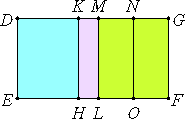# Proposition 64

The square on the side of a rational plus a medial area applied to a rational straight line produces as breadth the fifth binomial.

Let AB be the side of a rational plus a medial area, divided into its straight lines at C, so that AC is the greater, let a rational straight line DE be set out, and let there be applied to DE the parallelogram DF equal to the square on AB, producing DG as its breadth.

I say that DG is a fifth binomial straight line.

Make the same construction as before.X.40

Since AB is the side of a rational plus a medial area, divided at C, therefore AC and CB are straight lines incommensurable in square which make the sum of the squares on them medial but the rectangle contained by them rational.

X.22

Since, then, the sum of the squares on AC and CB is medial, therefore DL is medial, so that DM is rational and incommensurable in length with DE.

X.20

Again, since twice the rectangle AC by CB, that is MF, is rational, therefore MG is rational and commensurable with DE.

Therefore DM is incommensurable with MG. Therefore DM and MG are rational straight lines commensurable in square only. Therefore DG is binomial.

I say next that it is also a fifth binomial straight line.

X.18

For it can be proved similarly that the rectangle DK by KM equals the square on MN, and that DK is incommensurable in length with KM. Therefore the square on DM is greater than the square on MG by the square on a straight line incommensurable with DM.

And DM and MG are commensurable in square only, and the less, MG, is commensurable in length with DE.

Therefore DG is a fifth binomial.

Therefore, the square on the side of a rational plus a medial area applied to a rational straight line produces as breadth the fifth binomial.

Q.E.D.

## Guide

This proposition is used in X.72.